Home    |    Teacher    |    Parents    |    Glossary    |    About UsUse the number line for adding and subtracting integers:

• Add a positive integer by moving to the right on the number line
• Add a negative integer by moving to the left on the number line
• Subtract an integer by adding its opposite

Try it by clicking on the problems below.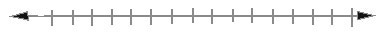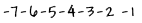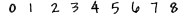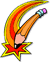Watch out! The negative of a negative is the opposite positive number. That is, for real numbers, - (- a ) = + a
 Homework Help | Pre-Algebra | NumbersEmail this page to a friendSearch·  Place value·  Decimal numbers·  Estimating and     rounding·  Adding / subtracting     decimals·  Multiplying decimals·  Dividing decimals·  Percent·  Exponents·  Square roots·  Signed integers·  Adding and     subtracting integers·  Multiplying and     dividing integers·  Properties of integers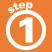First Glance In Depth Examples WorkoutAdding and Subtracting Integers